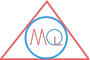MATHS QUERY

# Lines and Angles

## Relationship between Lines and Angles

We have learnt the basics of angles from its definition, measurement of an angle to its various types of angles. As we have seen that angles are formed only when two lines intersect at some point. So, there are many more case where different types of angles are defined when lines intersect each other.

Such angles are formed by a transversal lines when it intersects two or more parallel or non parallel lines.

## Vertically Opposite angles

When two straight lines intersect each other, they form four angles at the point of intersection. Out of four angles the two angles which are directly opposite to each other are called vertically opposite angles. These two vertically opposite angles are always equal.

Example of Vertically Opposite anglesHere, in the above diagram, we can see $$\angle AOD$$, $$\angle BOC$$, $$\angle AOC$$ and $$\angle BOD$$ are the four angles formed at point O when two lines AB and CD intersect at point O.

The angles $$\angle AOD$$ and $$\angle BOC$$ are directly opposite to each other, therefore they are called vertically opposite angles and $$\angle AOD = \angle BOC$$

Similarly, the angles $$\angle AOC$$ and $$\angle BOD$$ are also directly opposite to each other, therefore they are also called vertically opposite angles and $$\angle AOC = \angle BOD$$

Moreover, sum of each pair of adjacent angles is alway equal to $$180^0$$.

$$\angle AOC + \angle BOC = 180^0$$

$$\angle AOC + \angle AOD = 180^0$$

$$\angle BOC + \angle BOD = 180^0$$

$$\angle AOD + \angle BOD = 180^0$$

## Exterior angles

Exterior angles are formed at points on the exterior sides of the two lines where a transversal line intersects these two lines.

Let’s understand it by an example.

Example of Exterior angles$$\angle 1$$, $$\angle 2$$, $$\angle 7$$ and $$\angle 8$$ are formed at points A and B on the exterior sides of two non parallel lines l and m respectively, when a transversal line n cuts through them. Therefore, $$\angle 1$$, $$\angle 2$$, $$\angle 7$$ and $$\angle 8$$ are called as exterior angles.

## Interior angles

Interior angles are formed at points on the interior sides of the two lines where a transversal line intersects these two lines.

Let’s understand it by an example.

Example of Interior angles$$\angle 3$$, $$\angle 4$$, $$\angle 5$$ and $$\angle 6$$ are formed at points A and B on the interior sides of two non parallel lines l and m respectively, when a transversal line n cuts through them. Therefore, $$\angle 3$$, $$\angle 4$$, $$\angle 5$$ and $$\angle 6$$ are called as interior angles.

## Alternate angles

Alternate angles again are formed when a transversal line cuts through two parallel or non parallel lines. These angles are a pair of angles which can exist on the interior or exterior sides of the two lines.

We can understand it more precisely with the following example.

Example of Alternate anglesHere, a transversal line n cuts thru two lines l and m and forms total of eight angles at points A and B which are:

$$\angle 1$$, $$\angle 2$$, $$\angle 3$$, $$\angle 4$$, $$\angle 5$$, $$\angle 6$$, $$\angle 7$$ and $$\angle 8$$.

If we select the pairs of angles in the following way, then these pairs are the alternate angles.

$$\angle 1$$ and $$\angle 7$$

$$\angle 2$$ and $$\angle 8$$

$$\angle 3$$ and $$\angle 6$$

$$\angle 4$$ and $$\angle 5$$

Depending upon where the pair exists on the two lines l and m we can name them alternate interior angles and alternate exterior angles.

e.g. here, the pairs of alternate angles $$\angle 1$$ and $$\angle 7$$, $$\angle 2$$ and $$\angle 8$$ lie outside the lines i.e. exterior sides of the two lines, such type of alternate angles are called alternate exterior angles.

Similarly, the pairs of alternate angles $$\angle 3$$ and $$\angle 6$$, $$\angle 4$$ and $$\angle 5$$ lie inside the lines i.e. interior sides of the two lines, such type of alternate angles are called alternate interior angles.

Note

The pair of alternate angles formed with parallel lines are always equal.

In the above example, lines l and m are parallel lines. So, we can see that the following alternate angles pairs are equal

$$\angle 1 = \angle 7$$

$$\angle 2 = \angle 8$$

$$\angle 3 = \angle 6$$

$$\angle 4 = \angle 5$$

## Corresponding angles

A pair of angeles is called corresponding angles in which one arm of both angles is on the same side of transversal and their other arm are directed in same sense.

If lines l and m are parallel, then pair of corresponding angeles are equal.

Let’s understand it with the following example.

Example of Corresponding anglesSo, from the above diagram, the following pair of angles are corresponding angles that are formed on two parallel lines l and m.

$$\angle 1$$ and $$\angle 6$$

$$\angle 2$$ and $$\angle 5$$

$$\angle 3$$ and $$\angle 7$$

$$\angle 4$$ and $$\angle 8$$

As said above, these pair of angles, also called as corresponding angles, are equal always.

Therefore, we can write them as:

$$\angle 1 = \angle 6$$

$$\angle 2 = \angle 5$$

$$\angle 3 = \angle 7$$

$$\angle 4 = \angle 8$$

More, in parallel lines l and m, sum of interior angles of the same side of transversal is $$180^0$$ i.e. $$\angle 4$$ + $$\angle 6$$ = $$180^0$$

$$\angle 3$$ + $$\angle 5$$ = $$180^0$$

## Linear pair of angles

When sum of two adjacent angles is $$180^0$$, they are called linear pair of angles.

Or, we can say when supplementary angles formed on a straight line, those angles are called a linear pair of angles.

Example of Linear pair of anglesIn the above diagram, $$\angle ACD$$ + $$\angle BCD$$ = $$180^0$$, therefore, they form a linear pair of angles.